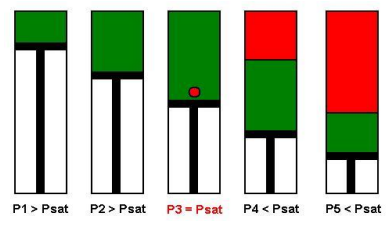## PVT Sampling Methods: Downhole, Wellhead & Surface Recombination

Samples of the reservoir fluid are usually collected at an early stage in the well’s producing life and dispatched to a laboratory for the f PVT analysis. The objectives of sampling are to receive samples from a suitable place in the production wells or surface facilities. The samples should represent the system in the reservoir under its initial conditions in order to determine its type, volumetric and phase behavior, and its composition. PVT analysis results are needed for geological and reservoir engineering evaluation and forecasting, as well as for laboratory studies concerning enhanced oil recovery (EOR).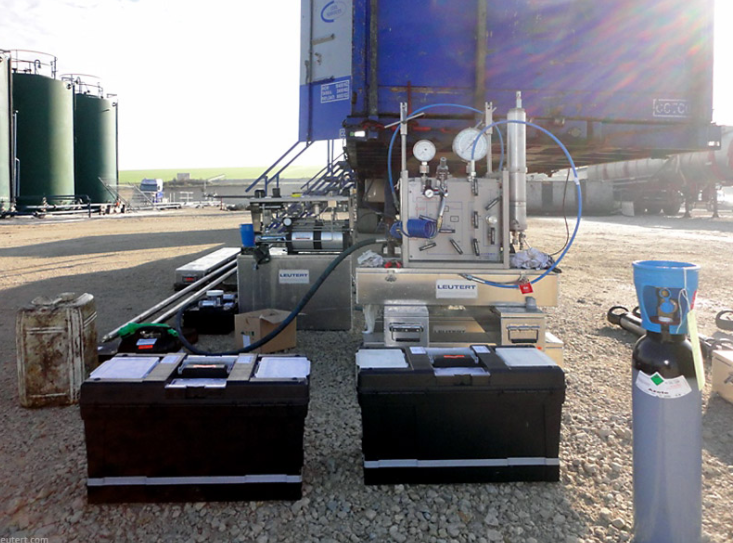There are basically three ways of collecting fluids samples from a well, either by Downhole SamplingWellhead Sampling or by  Surface Recombination Sampling.

## PVT Experiments: Separator Test

Separator Test experiments are carried out for both oil and gas condensate mixtures. A sample of reservoir liquid is placed in the laboratory cell and brought to reservoir temperature and bubble-point pressure. Then the liquid is expelled from the cell through a number of stages of separation.  Usually, two or three stages of separation are used, with the last stage at atmospheric pressure and near-ambient temperature (60 to 80°F).

The gas is let out of the separator through the top and is transferred to standard conditions, where its volume is measured. As for the differential liberation experiment, liquid dropping out from the gas is converted to an equivalent gas volume at standard conditions.

The liquid from the ﬁrst separator is let into a second separator at a lower pressure and temperature than the ﬁrst one. At which conditions, more gas will be liberated as sketched in the figure below. As with the gas from the ﬁrst separator, this gas is transferred to standard conditions.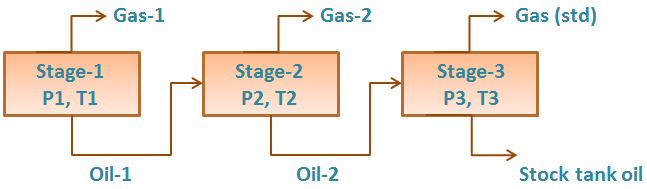The oil remaining after gas removal is brought to the conditions of the next separator stage. The gas is removed again and quantified by moles and specific gravity. Oil volume is noted, and the process is repeated until stock-tank conditions are reached. Final oil volume, Vo, and specific gravity, SGo, are measured at 60°F.

## PVT Experiments: Differential Liberation

The bubble point pressure is determined by an experiment called “Constant Composition Expansion” (CCE), also called: “flash liberation”. The device used to perform this experiment is the PV cell, as shown and described in the article “Constant Composition Expansion “. The Differential Liberation (DL), discussed in this article, is experimentally performed in a similar PV cell.

The main difference between these two types of experiments is that in the Constant Composition Expansion (or flash expansion) no gas is removed from the PV cell. But instead, the gas remains in equilibrium with the oil. As a result, the overall hydrocarbon composition in the cell remains unchanged.

In the differential liberation experiment, however, pressure gradually decreases in steps and any liberated gas is removed from the oil. All depletion stages are performed at the same reservoir temperature. Therefore, there is a continual compositional change in the PV cell, the remaining hydrocarbons becoming progressively richer in the heavier components, and the average molecular weight thus increasing.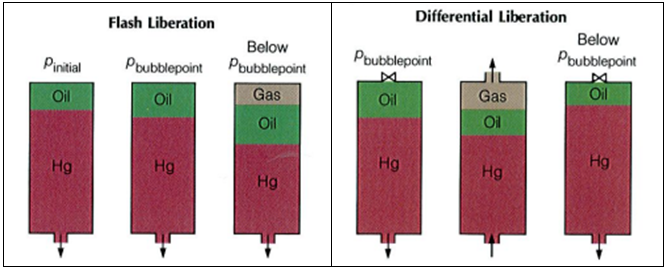The differential liberation experiment starts at the bubble point pressure determined from the CCE (since above this pressure the flash and differential experiments are identical).

### Example*:

The following example guides you on how to use and interpret the data from Differential Liberation test. The reservoir temperature is T= 200 °F and the bubble point pressure is 3330 psia.

The essential data obtained from the differential liberation experiment, performed on the same oil sample as CCE test, are listed in the following table:

## Effect of CO2 on downhole flowrate calculation

Downhole flow rate can be calculated from surface flow rate (stock tank barrels) using the following equation. It is assumed that no gas is dissolved in the water phase and the water formation volume factor is equal to one.

Downhole flow rate = [(Oil rate)sc × Bo] + [(Free GOR) × (Oil rate)sc × Gas FVF] + (Water rate)sc

Free GOR = Producing GOR – Solution GOR, therefore:

## q = ( Qo × Bo ) + [ ( R – Rs) × Qo × Bg × 1000] + Qw

Where:

• q = downhole flow rate (bbl/d or m3/d)
• Qo = Oil flow rate at standard conditions (stb/d or m3sc/d)
• Bo = Oil formation volume factor (bbl/stb or m3sc/m3sc)
• R = Producing gas-oil ratio (scf/stb or m3sc/m3sc)
• Rs = Solution gas-oil ration (scf/stb or m3sc/m3sc)
• Bg = Gas formation vol. factor (bbl/mscf or m3sc/m3sc)
• Qw = Water flow rate at standard conditions (stb/d or m3sc/d)

### Effect of CO2 on downhole flowrate calculation: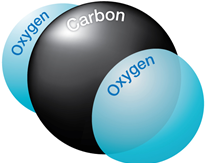If CO2 is present, the calculation of downhole flow rate becomes more complex for many reasons:

## PVT Experiments: Constant Composition Expansion

Once we have a sample of reservoir fluid, either directly or by recombination, we need to do reservoir fluid measurement, often called PVT analysis. There are mainly 6 fluid properties measured: oil density, gas density, solution GOR, bubble point pressure, formation volume factor, and viscosity. This post will discuss how the bubble point pressure is measured and what the Constant Composition Expansion Experiment is?

### Experimental apparatus:

The bubble point pressure is determined by an experiment called Constant Composition Expansion (CCE). It’s also called Constant Mass Expansion (CME) experiment or Pressure-Volume (PV) relationship.

The apparatus used to perform this experiment is the PV cell, as shown in the figure below. The fluid is charged in the PV cell after recombining the oil and gas in the correct proportions. The temperature, controlled by a thermostat, is maintained constant throughout the experiment (the measured reservoir temperature). The cell pressure is controlled by a positive displacement pump and recorded on an accurate pressure gauge.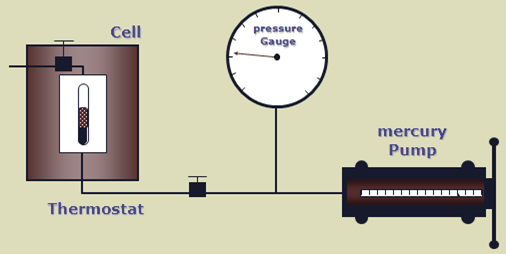### Bubble Point Pressure:

The bubble point pressure is the pressure, at some reference temperature, that the first bubble of gas is liberated from the liquid phase. It is also known as the saturation pressure.
The reference temperature is usually the reservoir temperature, but any temperature can be used.
Note that the bubble point pressure is a function of temperature and changing the reference temperature will change the bubble point pressure.

### Bubble Point Measurement:

A schematic of a Constant Composition Expansion experiment steps is shown in the figure below. The PV cell pressure is initially raised to a value greater than the bubble point pressure. the pressure is subsequently reduced in stages, and on each stage, the volume of the cell is recorded.